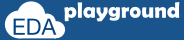# SystemC Statement and Flow Control

### Statements

All the statements will end with semicolon (;), Number of statements can be grouped by placing them with in curly braces. { };
```{
Statement1;
Statement2;
Statement3;
}```

### if and else

The if  keyword is used to execute the statement or group of statements based on the condition’s.

Below are the few examples,

`//if, execution of one statement on condition satisfied`
```if ( condition ) statement;
```
```//if, execution of block/statement's on condition satisfied
if ( condition ) {
Statement1;
Statement2;
}
```
```//if along with else part
if(condition) {
Statement1;
}
else {
stetement2;
}
```
```//if, with nested if in else part
if(condition2) {
Statement1;
}
else  if (condition2) {
if(condition3)  stetement2;
else            statement3;
}```

#### Example-1:

```#include "systemc.h"
int sc_main (int argc, char* argv[]) {
//declaration and initialization
int a = 10;
int b = 30;

//if-else
if( a > b )
cout <<" a is greater than b "<<endl;
else
{
cout <<" b is greater than a "<<endl;
if((b-a) > a)
cout <<" b-a is greater than a"<<endl;
else
cout <<" b-a is less than a "<<endl;
}

// Terminate simulation
return 0;
}
```

#### Simulator Output:

b is greater than a
b-a is greater than a

### Loop Statements:

Below are the loop statements in C++/SystemC,

• For Loop
• While Loop
• Do-while

#### for loop

for (initialization; condition; increment/decrement) statement;
for loop works as below,
• Initialize the loop variable
• Check for the condition, if condition is satisfied then executes the block
• Increment/decrement the loop variable value

#### Example-1: for-loop

```#include "systemc.h"
int sc_main (int argc, char* argv[]) {

//for-loop
for(int i=0; i<4; i++)
{
cout <<" Value of i = "<<i<<endl;
}

// Terminate simulation
return 0;
}
```

#### Simulator Output:

Value of i = 0
Value of i = 1
Value of i = 2
Value of i = 3
Execute the above code on#### Example-2: for-loop, one or more initial declaration

```#include "systemc.h"
int sc_main (int argc, char* argv[]) {

//for-loop
for ( int j=0,i=3;j<5;j++)
{
if(j==i)
cout <<" Value j equals to Value of i. j="<<j<<" i="<<i<<endl;
else
cout <<" Value j ="<<j<<endl;
}

// Terminate simulation
return 0;
}
```

#### Simulator Output:

Value j =0
Value j =1
Value j =2
Value j equals to Value of i. j=3 i=3
Value j =4
Execute the above code on#### Example-3: for-loop, one or more step assignment

```#include "systemc.h"
int sc_main (int argc, char* argv[]) {

//for-loop
for ( int j=0,i=4;j<4;j++,i--)
{
cout <<" j="<<j<<" i="<<i<<endl;
}

// Terminate simulation
return 0;
}
```

#### Simulator Output:

j=0 i=4
j=1 i=3
j=2 i=2
j=3 i=1
Execute the above code on#### While Loop

The while loop will repeats the execution of statement/block until the condition is true.

#### Example-1: while-loop

```#include "systemc.h"
int sc_main (int argc, char* argv[]) {
//declaration and initialization
int a = 15;
int b = 10;

//while-loop
while( a > b ) {
cout <<" a is greater than b a = "<<a<<" b = "<<b<<endl;
a--;
}

cout <<" b is greater than a "<<endl;

// Terminate simulation
return 0;
}
```

#### Simulator Output:

a is greater than b a = 15 b = 10
a is greater than b a = 14 b = 10
a is greater than b a = 13 b = 10
a is greater than b a = 12 b = 10
a is greater than b a = 11 b = 10
b is greater than a
Execute the above code on#### Do-while

The do-while loop will execute the statement/block and then checks for condition.
The difference between do-while and while is, irrespective of the condition the statement/block will be executed at least once in do-while.

#### Example-1: do-while

```#include "systemc.h"
int sc_main (int argc, char* argv[]) {
//declaration and initialization
int a = 9;
int b = 10;

//while-loop
do{
cout <<" a is greater than b a = "<<a<<" b = "<<b<<endl;
a--;
}while( a > b );

cout <<" b is greater than a "<<endl;

// Terminate simulation
return 0;
}
```

#### Simulator Output:

a is greater than b a = 9 b = 10
b is greater than a
Execute the above code on❮ Previous Next ❯